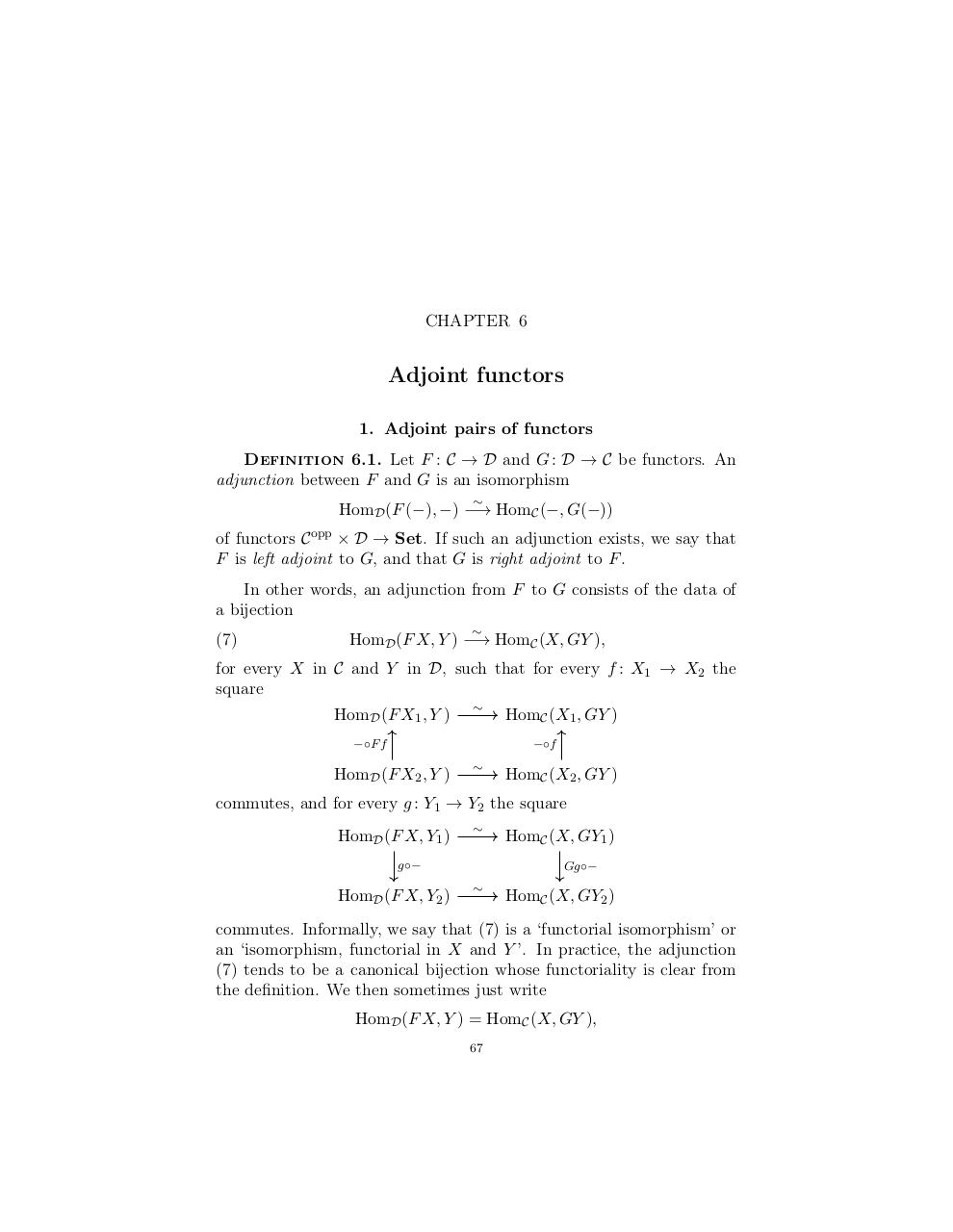# yoneda lenny.pdfPage 1 2 3 4 5 6 7 8 9

#### Text preview

CHAPTER 6

Definition 6.1. Let F : C → D and G : D → C be functors. An
adjunction between F and G is an isomorphism

HomD (F (−), −) −→ HomC (−, G(−))
of functors C opp × D → Set. If such an adjunction exists, we say that
F is left adjoint to G, and that G is right adjoint to F .
In other words, an adjunction from F to G consists of the data of
a bijection
(7)

HomD (F X, Y ) −→ HomC (X, GY ),

for every X in C and Y in D, such that for every f : X1 → X2 the
square
HomD (F X1 , Y )

−◦F f

HomC (X1 , GY )
−◦f

HomD (F X2 , Y )

HomC (X2 , GY )

commutes, and for every g : Y1 → Y2 the square
HomD (F X, Y1 )

g◦−

HomD (F X, Y2 )

HomC (X, GY1 )
Gg◦−

HomC (X, GY2 )

commutes. Informally, we say that (7) is a ‘functorial isomorphism’ or
an ‘isomorphism, functorial in X and Y ’. In practice, the adjunction
(7) tends to be a canonical bijection whose functoriality is clear from
the definition. We then sometimes just write
HomD (F X, Y ) = HomC (X, GY ),
67